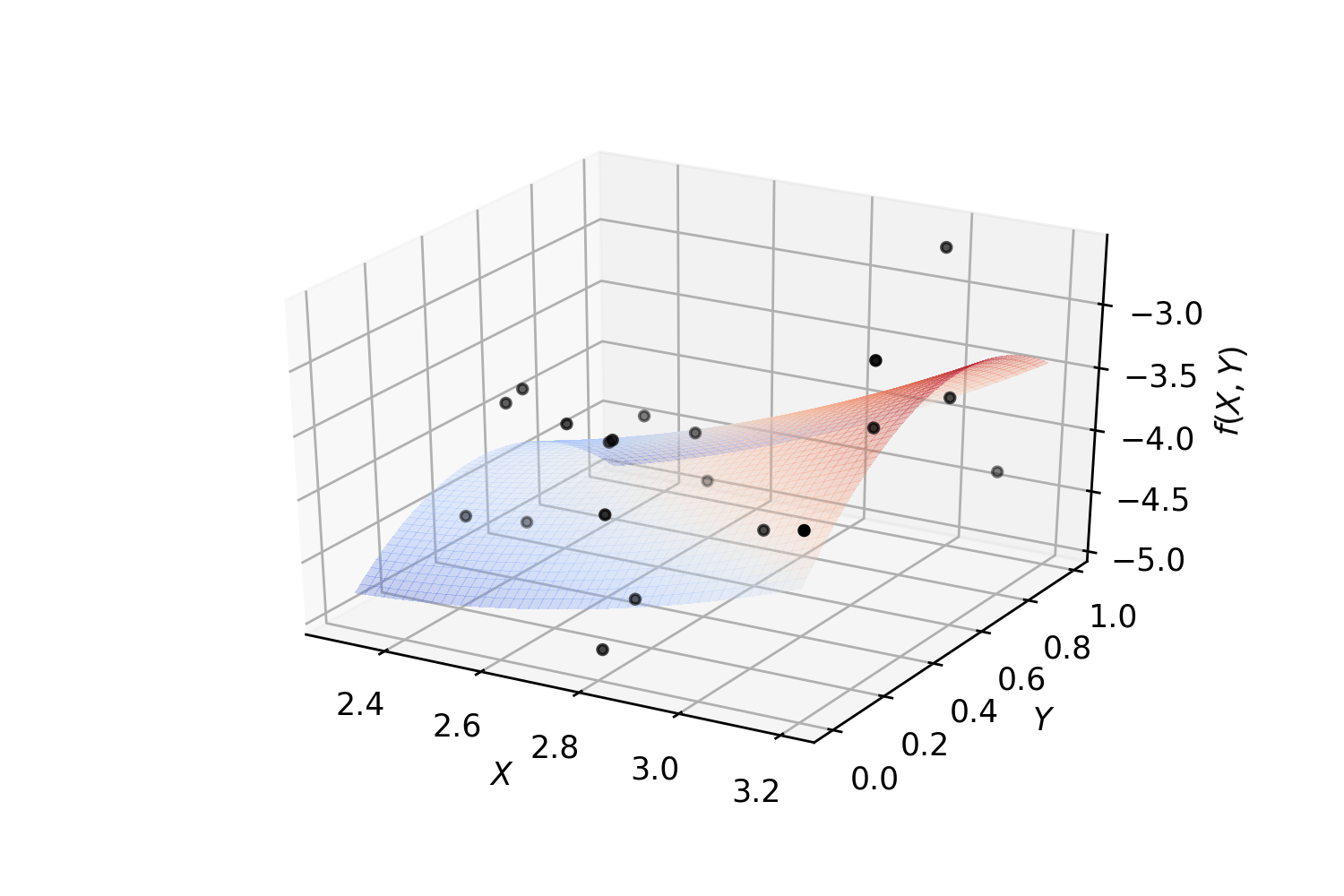# Hilbert Spaces¶

## Orthonormal system of functions¶

Let X be a topological space and $$\mu$$ be a finite Borel measure on X. The bilinear function $$\langle\cdot,\cdot\rangle$$ defined on $$L_2(X, \mu)$$ as $$\langle f, g\rangle = \int_X fg d\mu$$ is an inner product which turns $$L_2(X, \mu)$$ into a Hilbert space.

Let us denote the family of all continuous real valued functions on a non-empty compact space X by $$\textrm{C}(X)$$. Suppose that among elements of $$\textrm{C}(X)$$, a subfamily A of functions are of particular interest. Suppose that A is a subalgebra of $$\textrm{C}(X)$$ containing constants. We say that an element $$f\in\textrm{C}(X)$$ can be approximated by elements of A, if for every $$\epsilon>0$$, there exists $$p\in A$$ such that $$|f(x)-p(x)|<\epsilon$$ for every $$x\in X$$. The following classical results guarantees when every $$f\in\textrm{C}(X)$$ can be approximated by elements of A.

Let $$(V, \langle\cdot,\cdot\rangle)$$ be an inner product space with $$\|v\|_2=\langle v,v\rangle^{\frac{1}{2}}$$. A basis $$\{v_{\alpha}\}_{\alpha\in I}$$ is called an orthonormal basis for V if $$\langle v_{\alpha},v_{\beta}\rangle=\delta_{\alpha\beta}$$, where $$\delta_{\alpha\beta}=1$$ if and only if $$\alpha=\beta$$ and is equal to 0 otherwise. Every given set of linearly independent vectors can be turned into a set of orthonormal vectors that spans the same sub vector space as the original. The following well-known result gives an algorithm for producing such orthonormal from a set of linearly independent vectors:

Note

Gram–Schmidt

Let $$(V,\langle\cdot,\cdot\rangle)$$ be an inner product space. Suppose $$\{v_{i}\}^{n}_{i=1}$$ is a set of linearly independent vectors in V. Let

$u_{1}:=\frac{v_{1}}{\|v_{1}\|_2}$

and (inductively) let

$w_{k}:=v_{k}-\sum_{i=1}^{k-1}\langle v_{k},u_{i}\rangle u_{i}\textrm{ and } u_{k}:=\frac{w_{k}}{\|w_{k}\|_2}.$

Then $$\{u_{i}\}_{i=1}^{n}$$ is an orthonormal collection, and for each k,

$span\{u_{1},u_{2},\cdots,u_{k}\}=span\{v_{1},v_{2},\cdots,v_{k}\}.$

Note that in the above note, we can even assume that $$n=\infty$$.

Let $$B=\{v_1, v_2, \dots\}$$ be an ordered basis for $$(V,\langle\cdot,\cdot\rangle)$$. For any given vector $$w\in V$$ and any initial segment of B, say $$B_n=\{v_1,\dots,v_n\}$$, there exists a unique $$v\in\textrm{span}(B_n)$$ such that $$\|w-v\|_2$$ is the minimum:

Note

Let $$w\in V$$ and B a finite orthonormal set of vectors (not necessarily a basis). Then for $$v=\sum_{u\in B}\langle u,w\rangle u$$

$\|w-v\|_2 = \min_{z\in\textrm{span}(B)}\|w-z\|_2.$

Now, let $$\mu$$ be a finite measure on X and for $$f,g\in\textrm{C}(X)$$ define $$\langle f,g\rangle=\int_Xf g d\mu$$. This defines an inner product on the space of functions. The norm induced by the inner product is denoted by $$\|\cdot\|_{2}$$. It is evident that

$\|f\|_{2}\leq\|f\|_{\infty}\mu(X),~\forall f\in\textrm{C}(X),$

which implies that any good approximation in $$\|\cdot\|_{\infty}$$ gives a good $$\|\cdot\|_{2}$$-approximation. But generally, our interest is the other way around. Employing Gram-Schmidt procedure, we can find $$\|\cdot\|_{2}$$ within any desired accuracy, but this does not guarantee a good $$\|\cdot\|_{\infty}$$-approximation. The situation is favorable in finite dimensional case. Take $$B=\{p_1,\dots,p_n\}\subset\textrm{C}(X)$$ and $$f\in\textrm{C}(X)$$, then there exists $$K_f>0$$ such that for every $$g\in\textrm{span}(B\cup\{f\})$$,

$K_f\|g\|_{\infty}\leq\|g\|_{2\leq}\|g\|_{\infty}\mu(X).$

Since X is assumed to be compact, $$\textrm{C}(X)$$ is separable, i.e., $$\textrm{C}(X)$$ admits a countable dimensional dense subvector space (e.g. polynomials for when X is a closed, bounded interval). Thus for every $$f\in\textrm{C}(X)$$ and every $$\epsilon>0$$ one can find a big enough finite B, such that the above inequality holds. In other words, good enough $$\|\cdot\|_{2}$$-approximations of f give good $$\|\cdot\|_{\infty}$$-approximations, as desired.

Example. Polynomial regression on 2-dimensional random data:

from mpl_toolkits.mplot3d import Axes3D
from matplotlib import cm
import matplotlib.pyplot as plt
import numpy as np
from SKSurrogate.NpyProximation import HilbertRegressor, FunctionBasis
def randrange(n, vmin, vmax):
'''
Helper function to make an array of random numbers having shape (n, )
with each number distributed Uniform(vmin, vmax).
'''
return (vmax - vmin)*np.random.rand(n) + vmin

# degree of polynomials
deg = 2
FB = FunctionBasis()
B = FB.Poly(2, deg)
# initiate regressor
regressor = HilbertRegressor(base=B)
# number of random points
n = 20
fig = plt.figure()
ax = fig.add_subplot(111, projection='3d')
for c, m, zlow, zhigh in [('k', 'o', -5, -2.5)]:
xs = randrange(n, 2.3, 3.2)
ys = randrange(n, 0, 1.0)
zs = randrange(n, zlow, zhigh)
ax.scatter(xs, ys, zs, c=c, s=10, marker=m)
ax.set_xlabel('$X$')
ax.set_ylabel('$Y$')
ax.set_zlabel('$f(X,Y)$')
X = np.array([np.array((xs[_], ys[_])) for _ in range(n)])
y = np.array([np.array((zs[_],)) for _ in range(n)])
X_ = np.arange(2.3, 3.2, 0.02)
Y_ = np.arange(0, 1.0, 0.02)
_X, _Y = np.meshgrid(X_, Y_)
# fit the regressor
regressor.fit(X, y)
# prepare the plot
Z = []
for idx in range(_X.shape):
_X_ = _X[idx]
_Y_ = _Y[idx]
_Z_ = []
for jdx in range(_X.shape):
t = np.array([np.array([_X_[jdx], _Y_[jdx]])])
_Z_.append(regressor.predict(t))
Z.append(np.array(_Z_))
Z = np.array(Z)
surf = ax.plot_surface(_X, _Y, Z, cmap=cm.coolwarm, linewidth=0, antialiased=False, alpha=.3)